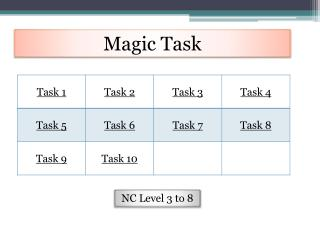DownloadDownload PresentationDownload Presentation- - - - - - - - - - - - - - - - - - - - - - - - - - - E N D - - - - - - - - - - - - - - - - - - - - - - - - - - -
##### Presentation Transcript

1. Magic Task NC Level 3 to 8

2. Magic Task 1 Fill in the numbers to make the sum of each row, column and diagonal equal to 15. Use 2, 5, 7, 9 Use 1, 2, 6, 7, 9 http://nrich.maths.org/969 Home

3. Magic Task 2 Arrange three 1s, three 2s and three 3s in this square so that every row, column and diagonal adds to the same total. http://nrich.maths.org/1819 Home

4. Magic Task 3 Fill in the numbers to make the sum of each row, column and diagonal equal to 34 Use 2, 7, 8, 12, 13, 14 Use 1, 2, 5, 6, 11, 12, 15, 16 http://nrich.maths.org/970 Home

5. Magic Task 4 The rows, columns and main diagonals of a 1 - 9 magic square add to the same number, 15. This magic square has had doors, with operations written in them, opened to make it into a maze Start wherever you like and go through every cell using all the numbers, doing the operation indicated to the numbers, and go out through the exit marked in cell 8 with a total of 15! You must use the operations and numbers strictly in the order you meet them http://nrich.maths.org/1072 Home

6. http://nrich.maths.org/87 Magic Task 5 • This is a 4 x 4 Magic Square made from the numbers 1 to 16. • In a Magic Square all the rows, columns and diagonals add to a number called the 'Magic Constant'. • What is the Magic Constant of this • Magic Square? • What happens to the Magic constant • If you add 2 to each number? x2? -1? • Create a magic square with a Magic constant of 18 • What other numbers below 100 can you make into a Magic constants by changing each of the numbers in the same way? • Are there some numbers that can’t be made Home

7. Magic Task 6 Complete the magic square using the numbers 1 to 25 once each.Each row, column and diagonal adds up to 65.Each square is identified by its column and row: Here are the clues: Perfect squares are in b5, b3, d3, b1 and c1.Prime numbers are in a5, c5, e5, c4, a3, c3, e3, e2 and a1.Triangular numbers are in d5, e4, d3, a1, e1 and c2.Perfect cubes are in d3 and b2.Powers of 2 are in b5, b2, e2 and b1.Palindromic numbers are in a5 and d1.Factors of 100 are in b5, d5, c4, b3, d3, a2 and e2.The median of all the numbers is in c3.Row 3 and column c are all odd.Numbers that are the same upside-down are in a5, d3 and b2. http://nrich.maths.org/1187 Home

8. Magic Task 7 Choose a number and place it in the centre of the blank “magic” square. Use the expressions shown in the diagram to generate the other 8 numbers. Check that it is a magic square Add the expressions to prove it is always a magic square. Can you generate other expressions that generate magic squares? Home

9. Magic Task 8 Complete this magic square by ensuring that the sum of each row and column and diagonal is equal. Choose any number for n and substitute it into the Expressions to produce a set of 9 numbers Can you generate other magic squares using expressions like The one shown Home

10. Magic Task 9 Complete this magic square by ensuring that the sum of each row and column is equal. Choose any numbers for a and p and k and substitute it into the expressions to produce a Magic Square Home

11. Magic Task 10 Can you show that the sum of a magic square is always three times the number in the centre. Can you prove it? Make all of the magic squares that you can using the fractions 7/6, 4/3 3/2. Home